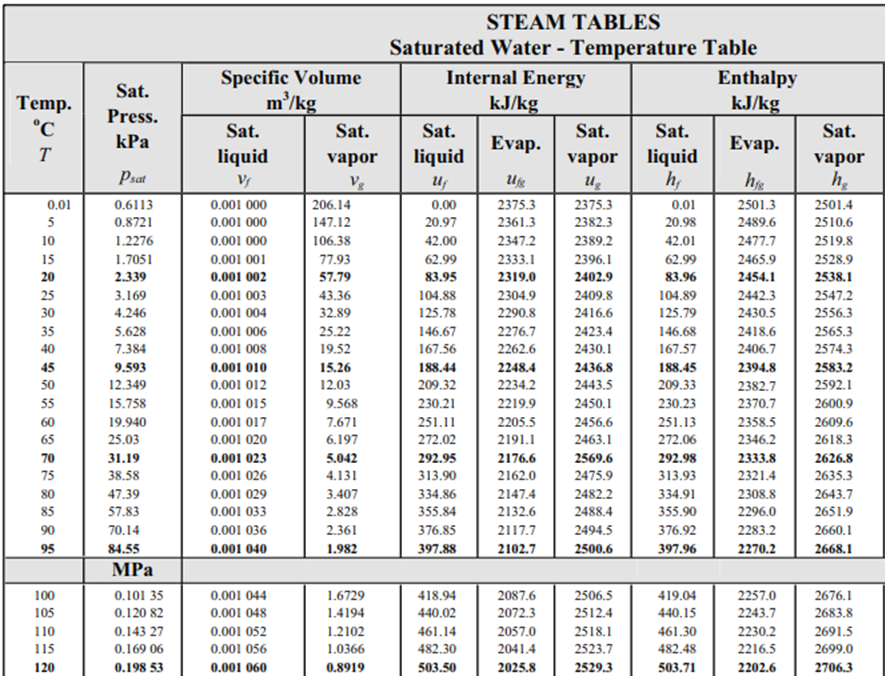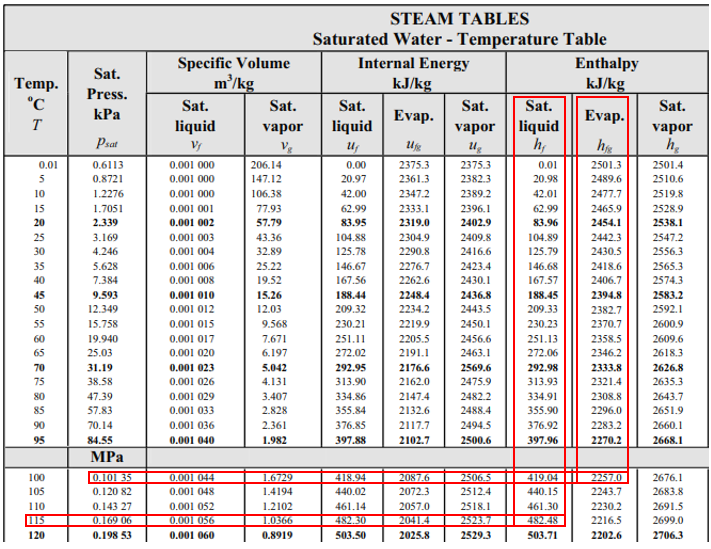## Steam Tables

Saturated water at 115°C flows into a throttling valve, and exits the valve at 0.10135 MPa. At exit, what is the quality of the water?Hint
Quality, x, (for two-phase liquid-vapor systems at saturation) is defined as
$$h=xh_g+(1-x)h_f\:or\:h=h_f+xh_{fg}$$$where $$h_{fg}$$ is the enthalpy change for phase transitions (evaporation), $$h_f$$ is the enthalpy of saturated liquid, $$h_g$$ is the enthalpy for saturated vapor, and $$h$$ is specific enthalpy. Hint 2Quality, x, (for two-phase liquid-vapor systems at saturation) is defined as $$h=xh_g+(1-x)h_f\:or\:h=h_f+xh_{fg}$$$
where $$h_{fg}$$ is the enthalpy change for phase transitions (evaporation), $$h_f$$ is the enthalpy of saturated liquid, $$h_g$$ is the enthalpy for saturated vapor, and $$h$$ is specific enthalpy.
From the steam table at 115°C, the saturation pressure is 0.16906 MPa, and the enthalpy, $$h_1$$ , is 482.48 kJ/kg (the problem states saturated water at 115°C). Because enthalpy does not change as liquid passes through a throttling valve, $$h_1=h_2=482.48\:kJ/kg$$ . In the steam table again, 0.1035 MPa correlates to $$T=100°C$$ , $$h_f=419.04\:kJ/kg$$ and $$h_{fg}=2257\:kJ/kg$$ .Thus, for our problem statement:
$$h_1=h_f+xh_{fg}$$$$$482.48\frac{kJ}{kg}=419.04\frac{kJ}{kg}+x(2257\frac{kJ}{kg})$$$
$$x=\frac{63.44}{2257}=0.028$$\$
0.028